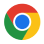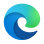# 3DOptix Blog

## Unlocking the World of Optics: Angle of Incidence – Definition, Formula, and Applications

#### Introduction

In the fascinating realm of physics and optical engineering, the angle of incidence is a fundamental concept that serves as a cornerstone for understanding how light interacts with surfaces and materials. In this comprehensive guide, tailored for the discerning audience of Ph.D. physicists and optical engineers, we will delve deep into the concept of the angle of incidence, exploring its definition, formula, practical examples, and its pivotal role in optics.

#### Angle of Incidence - The Basics

Defining the Angle of Incidence: The angle of incidence, symbolized as “{i}“, stands at the forefront of optics, representing the angle formed between an incident ray of light and the normal (perpendicular) to the surface it encounters. Essentially, it signifies the angle at which light strikes a surface, a crucial parameter in the world of optics. Mathematically, the angle of incidence is defined by the following formula:
{i} = \arctan(\frac{h}{d})

Where:

{i} = Angle of incidence.

{h} = Height at which the ray strikes the surface.

{d} = Distance between the point of incidence and the point where the normal intersects the surface.

Explanation of the Formula: The formula for the angle of incidence involves the arctan function because it calculates the angle formed by the ratio of the height at which the ray strikes the surface ({h}) to the distance ({d}) from the point of incidence to where the normal line intersects the surface. This mathematical expression provides a precise measurement of the angle at which light approaches the surface.

#### Relation to Angle of Reflection and Refraction

Understanding the angle of incidence is intrinsically tied to the angles of reflection and refraction. When light encounters a surface, several intriguing phenomena unfold:
• Reflection: A portion of the incident light reflects off the surface, obeying the law of reflection. The angle of reflection ({r}) is always equal to the angle of incidence ({i}), creating a profound connection: {i}={r}.
Explanation of the Law of Reflection: The law of reflection states that the angle at which light approaches a surface (angle of incidence) is equal to the angle at which it bounces off the surface (angle of reflection). This fundamental principle is the basis for the creation of accurate reflections in optical devices and is crucial in understanding how mirrors work.

#### Relation to Angle of Refraction and Snell's Law

The angle of incidence also plays a vital role in the phenomenon of refraction. Refraction occurs when light passes from one medium to another, such as from air to water or from glass to air. Snell’s Law governs this behavior and relates the angle of incidence ({i}) to the angle of refraction ({t}).

Explanation of Snell’s Law: Snell’s Law states that the ratio of the sine of the angle of incidence ({i}) to the sine of the angle of refraction ({t}) is constant for a given pair of media.
Mathematically, it is expressed as \frac{\sin{i}}{\sin{t}} = constant. This law is essential for understanding how light bends and changes direction when transitioning between different media, such as when a pencil appears bent in a glass of water.

Practical Applications of TIR: Total internal reflection finds diverse applications across various fields:
• Fiber Optics: In the world of telecommunications, optical fibers rely on total internal reflection to transmit data signals over long distances. Light is continually reflected within the fiber core, ensuring minimal signal loss and high-speed data transmission.
• Prisms: Optical prisms, such as those used in binoculars and spectrometers, exploit TIR to redirect and separate light. The critical angle determines the prism’s ability to refract or reflect light at specific angles.
• Diamonds: The exquisite brilliance of diamonds is partly attributed to TIR. Light entering a diamond reflects internally, enhancing its sparkle and allure.
• Underwater Photography: Photographers often employ the concept of TIR in underwater photography. A ring flash, positioned around the camera’s lens, utilizes TIR to provide even illumination in aquatic environments.

#### Practical Applications of the Angle of Incidence

The understanding of the angle of incidence extends far beyond theory, finding its practical utility in a multitude of real-world scenarios:
• Mirrors: The concept that the angle of incidence always equals the angle of reflection is instrumental in producing precise reflections in optical devices, telescopes, and periscopes. This knowledge enables engineers and designers to create accurate mirror systems for diverse applications, from automotive rearview mirrors to complex astronomical telescopes.
• Lenses: The angle of incidence plays a pivotal role in lens design and optical systems. Understanding how light bends and refracts when passing through lenses is critical for designing cameras, microscopes, eyeglasses, and other optical devices. Optical engineers use this knowledge to optimize the performance of these systems.
• Fiber Optics: The angle of incidence governs the propagation of light signals in optical fibers, which are essential in modern communication systems. By controlling the angle of incidence, engineers can efficiently transmit data over long distances with minimal signal loss. This technology underpins high-speed internet, telecommunication networks, and cable television.
• Astronomy: Astronomers rely on precise knowledge of the angle of incidence to study celestial objects. Telescopes and observatories use carefully designed mirrors and lenses to capture and focus light from distant stars, planets, and galaxies. Understanding how light behaves at different angles of incidence is essential for accurate astronomical observations.
• Medical Imaging: In medical imaging technologies like X-ray machines and endoscopes, the angle of incidence is crucial for capturing clear and detailed images. Medical professionals depend on precise imaging to diagnose and treat patients effectively.

#### Conclusion: Illuminating the World of Optics

In the captivating universe of optics, the angle of incidence shines as a guiding light, illuminating our understanding of how light behaves and interacts with surfaces and materials. As we conclude our exploration of this fundamental concept, let’s recap its pivotal role and significance.

The angle of incidence, denoted as “{i}“, serves as a foundational parameter, representing the angle at which an incident ray of light strikes a surface in relation to the normal line.

Mathematically expressed as {i} = \arctan(\frac{h}{d}), it allows us to precisely measure and analyze the behavior of light.

This angle is intrinsically connected to the angles of reflection and refraction, forming the basis for the laws of reflection and Snell’s Law. In reflection, the angle of incidence ({i}) equals the angle of reflection ({r}), a principle fundamental in creating accurate reflections in optical devices. In refraction, Snell’s Law relates the angle of incidence ({i}) to the angle of refraction ({t}), dictating how light bends when transitioning between different media.

Total internal reflection (TIR) introduces a critical angle ({C}) that leads to the captivating phenomenon where light remains entirely within a denser medium, finding applications in fiber optics, prisms, diamonds, and underwater photography.

Beyond theory, the angle of incidence finds practical utility in an array of real-world scenarios. From mirrors and lenses to fiber optics, astronomy, and medical imaging, this concept guides engineers and scientists in creating cutting-edge technologies that shape our modern world.

As we bid adieu to this journey through the angle of incidence, we invite you to continue exploring the intricate world of optics, where the interplay of light, surfaces, and angles continues to unlock new discoveries and innovations.## 3DOptix worksonly on desktop!

Please go to 3doptix.com on a
desktop device, using the
Chrome or Edge browser
••